Home | | RF and Microwave Engineering | Microwave Semiconductor Devices

# Microwave Semiconductor Devices

1. MICROWAVE SEMICONDUCTOR DEVICES 2. APPLICATION OF BJTS & FETS 3. PRICIPLE OF TUNNEL DIODE 4. VARACTOR AND STEP RECOVERY DIODE 5. TRANSFERRED ELECTRON DEVICES 6. GUNN DIODE 7. AVALANCE TRANSIT TIME DEVICES 8. IMPATT AND TRAPATT DIODE 9. PARAMETRIC DEVICES 10. APPLICATION OF PARAMETRIC DEVICES 11. MICROWAVE MONOLITHIC INTEGRATED CIRCUITES 12. METERIALS AND FABRICATION TECHNIQUES

MICROWAVE SEMICONDUCTOR DEVICES

1. MICROWAVE SEMICONDUCTOR DEVICES

OPERATION

Microwave solid-state devices are becoming increasingly important at microwave frequencies. These devices can be broken down into four groups. In the first group are the microwave bipolar junction transistor (BJT), the heterojunction bipolar transistor (HBT), and the tunnel diodes.

This group is discussed in this chapter. The second group includes microwave field-effect transistors (FETs) such as the junction field-effect transistors (JFETs), metal-semiconductor field-effect transistors (MESFETs), high electron mobility transistors (HEMTs), metal-oxide-semiconductor field-effect transistors (MOSFETs), the metal-oxide-semiconductor transistors and memory devices, and the charge-coupled devices ( CCDs).

This group is described in The third group, which is characterized by the bulk effect of the semiconductor, is called the transferred electron device (TED). These devices include the Gunn diode, limited space-charge-accumulation diode (LSA diode), indium phosphide diode (InP diode), and cadmium telluride diode ( CdTe diode).

This group is analyzed in Chapter 7. The devices of the fourth group, which are operated by the avalanche effect of the semiconductor, are referred to as avalanche diodes: the impact ionization avalanche transit-time diodes (IMPATT diodes), the trapped plasma avalanche triggered transit-time diodes (TRAPATT diodes), and the barrier injected transit-time diodes (BARITT diodes).

The avalanche diodes are studied in . All those microwave solid-state devices are tabulated in Table 5-0-1. In studying microwave solid-state devices, the electrical behavior of solids is the first item to be investigated. In this section it will be seen that the transport of charge through a semiconductor depends not only on the properties of the electron but also on the arrangement of atoms in the solids.

Semiconductors are a group of substances having electrical conductivities that are intermediate between metals and insulators. Since the conductivity of the semiconductors can be varied over wide ranges by changes in their temperature, optical excitation, and impurity content, they are the natural choices for electronic devices. The properties of important semiconductors are tabulated in Table 5-0-2. The energy bands of a semiconductor play a major role in their electrical behavior. For any semiconductor, there is a forbidden energy region in which no allowable states can exist.

The energy band above the forbidden region is called the conduction band, and the bottom of the conduction band is designated by Ec. The energy band below the forbidden region is called the valence band, and the top of the valence band is designated by Ev . The separation between the energy of the lowest conduction band and that of the highest valence band is called the bandgap energy E8 ,which is the most important parameter in semiconductors.

2. APPLICATION OF BJTS & FETS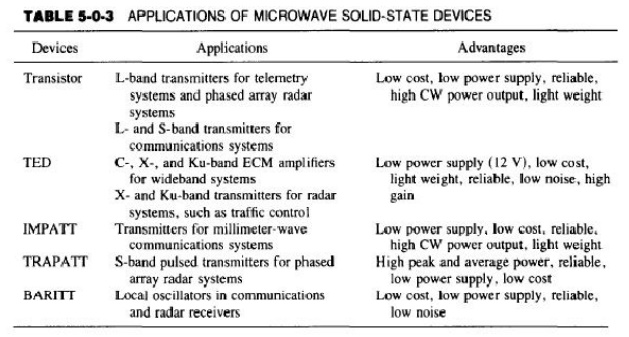3. PRICIPLE OF TUNNEL DIODE

MICROWAVE TUNNEL DIODES

Tunnel diodes are heavily doped PN junction diode that have a negative resistance over a portion of its V-I characteristics

Principles of Operation

The tunnel diode is a negative-resistance semiconductor p-n junction diode. The negative resistance is created by the tunnel effect of electrons in the p-n junction.

The doping of both the p and n regions of the tunnel diode is very high-impurity concentrations of 1019 to 1020 atoms/cm3 are used-and the depletion-layer barrier at the junction is very thin, on the order of 100 A or 10-6 em. Classically, it is possible for those particles to pass over the barrier if and only if they have an energy equal to or greater than the height of the potential barrier.

Quantum mechanically, however, if the barrier is less than 3 A there is an appreciable probability that particles will tunnel through the potential barrier even though they do not have enough kinetic energy to pass over the same barrier.

In addition to the barrier thinness, there must also be filled energy states on the side from which particles will tunnel and allowed empty states on the other side into which particles penetrate through at the same energy level.

In order to understand the tunnel effects fully, let us analyze the energy-band pictures of a heavily doped p-n diode.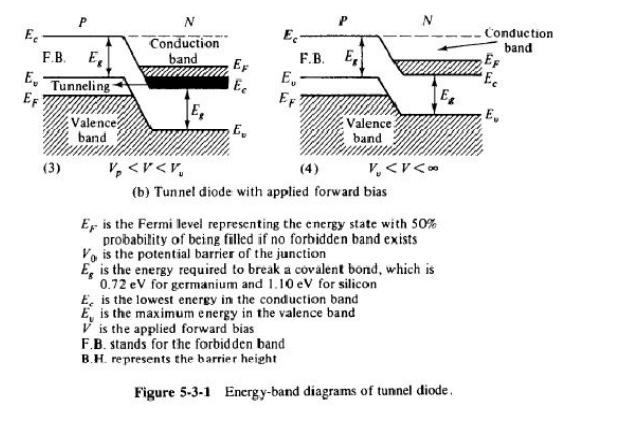Under open-circuit conditions or at zero-bias equilibrium, the upper levels of electron energy of both the type and n type are lined up at the same Fermi level as shown in Fig. 5-3-l(a).

Since there are no filled states on one side of the junction that are at the same energy level as empty allowed states on the other side, there is no flow of charge in either direction across the junction and the current is zero, as shown at point (a) of the volt-ampere characteristic curve of a tunnel diode in Fig.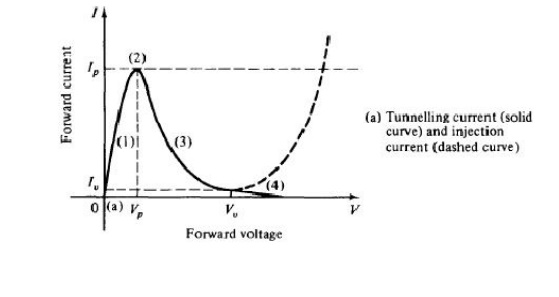In ordinary diodes the Fermi level exists in the forbidden band. Since the tunnel diode is heavily doped, the Fermi level exists in the valence band in p -type and in the conduction band inn-type semiconductors. When the tunnel diode is forwardbiased by a voltage between zero and the value that would produce peak tunneling current lp(O < V < Vp), the energy diagram is shown in part (1) of Fig. 5-3-l(b).

Accordingly, the potential barrier is decreased by the magnitude of the applied forward-bias voltage. A difference in Fermi levels in both sides is created.

Since there are filled states in the conduction band of the n type at the same energy level as allowed empty states in the valence band of the p type, the electrons tunnel through the barrier from the n type to the p type, giving rise to a forward tunneling current from thep type to then type as shown in sector (1) of Fig. 5-3-2(a). As the forward bias is increased to Vp, the picture of the energy band is as shown in part (2) of Fig. 5-3-l(b).

A maximum number of electrons can tunnel through the barrier from the filled states in the n type to the empty states in the p type, giving rise to the peak current Ip in Fig. 5-3-2(a). If the bias voltage is further increased, the condition shown in part (3) of Fig. 5-3-l(b) is reached.

The tunneling current decreases as shown in sector (3) of Fig. 5-3-2(a). Finally, at a very large bias voltage, the band structure of part (4) of Fig. 5-3-l(b) is obtained.

Since there are now no allowed empty states in the p type at the same energy level as filled states in the n type, no electrons can tunnel through the barrier and the tunneling current drops to zero as shown at point

(4) of Fig. 5-3-2(a).

When the forward-bias voltage V is increased above the valley voltage Vv , the ordinary injection current I at the p-n junction starts to flow.

This injection current is increased exponentially with the forward voltage as indicated by the dashed curve of Fig. 5-3-2(a). The total current, given by the sum of the tunneling current and the injection current, results in the volt-ampere characteristic of the tunnel diode as shown in Fig. 5-3-2(b).

It can be seen from the figure that the total current reaches a minimum value Iv (or valley current) somewhere in the region where the tunnel diode characteristic meets the ordinary p-n diode characteristic. The ratio of peak current to valley current (/p!Iv) can theoretically reach 50 to 100. In practice, however, this ratio is about 15.

4 . VARACTOR AND STEP RECOVERY DIODE

It is a high-efficiency microwave generator capable of operating from several hundred megahertz to several gigahertz. The basic operation of the oscillator is a semiconductor p-n junction diode reverse biased to current densities well in excess of those encountered in normal avalanche operation.

High-peak-power diodes are typically silicon n+ -p-p+ (or p+ -n-n+) structures with then-type depletion region width varying from 2.5 to 12.5 JLm. The doping of the depletion region is generally such that the diodes are well "punched through" at breakdown; that is, the de electric field in the depletion region just prior to breakdown is well above the saturated drift-velocity level. The device's p+region is kept as thin as possible at 2.5 to 7.5 JLm. The TRAPATT diode's diameter ranges from as small as 50 JLm for CW operation to 750 JLm at lower frequency for high peak- power devices.

Principles of Operation

high-field avalanche zone propagates through the diode and fills the depletion layer with a dense plasma of electrons and holes that become trapped in the low-field region behind the zone.

At point A the electric field is uniform throughout the sample and its magnitude is large but less than the value required for avalanche breakdown. The current density is expressed by

The current density is expressed bywhere Es is the semiconductor dielectric permittivity of the diode.

At the instant of time at point A, the diode current is turned on. Since the only charge carriers present are those caused by the thermal generation, the diode initially charges up like a linear capacitor, driving the magnitude of the electric field above the breakdown voltage.When a sufficient number of carriers is generated, the particle current exceeds the external current and the electric field is depressed throughout the depletion region, causing the voltage to decrease. This portion of the cycle is shown by the curve from point B to point C.

During this time interval the electric field is sufficiently large for the avalanche to continue, and a dense plasma of electrons and holes is created. As some of the electrons and holes drift out of the ends of the depletion layer, the field is further depressed and "traps" the remaining plasma.

The voltage decreases to point D. A long time is required to remove the plasma because the total plasma charge is large compared to the charge per unit time in the external current.

At pointE the plasma is removed, but a residual charge of electrons remains in one end of the depletion layer and a residual charge of holes in the other end. As the residual charge is removed, the voltage increases from point E to point F.

At point Fall the charge that was generated internally has been removed. This charge must be greater than or equal to that supplied by the external current; otherwise the voltage will exceed that at point A.

From point F to point G the diode charges up again like a fixed capacitor. At point G the diode current goes to zero for half a period and the voltage and the cycle repeats. The electric field can be expressed aswhere NA is the doping concentration of then region and xis the distance. Thus the value of t at which the electric field reaches Em at a given distance x into the depletion region is obtained by setting E(x, t) = Em, yielding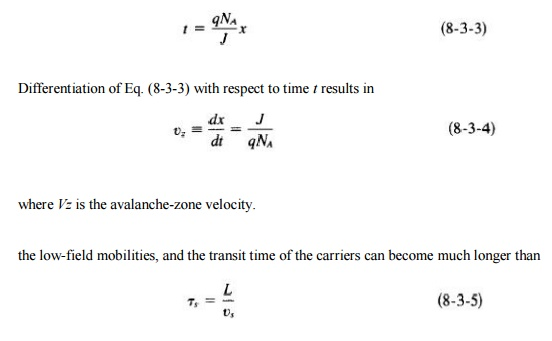Power Output and Efficiency

RF power is delivered by the diode to an external load when the diode is placed in a proper circuit with a load. The main function of this circuit is to match the diode effective negative resistance to the load at the output frequency while reactively terminating (trapping) frequencies above the oscillation frequency in order to ensure TRAPATT operation. To date, the highest pulse power of 1.2 kW has been obtained at 1.1 GHz (five diodes in series) , and the highest efficiency of 75% has been achieved at 0.6 GHz (11). Table 8-3-1 shows the current state of TRAPATT diodes

5. TRANSFERRED ELECTRON DEVICES

The application of two-terminal semiconductor devices at microwave frequencies has been increased usage during the past decades. The CW, average, and peak power outputs of these devices at higher microwave frequencies are much larger than those obtainable with the best power transistor. The common characteristic of all active two-terminal solid-state devices is their negative resistance. The real part of their impedance is negative over a range of frequencies. In a positive resistance the current through the resistance and the voltage across it are in phase. The voltage drop across a positive resistance is positive and a power of (/2 R) is dissipated in the resistance. In a negative resistance, however, the current and voltage are out of phase by 180°. The voltage drop across a negative resistance is negative, and a power of (-/ 2 R) is generated by the power supply associated with the negative resistance. In other words, positive resistances absorb power (passive devices), whereas negative resistances generate power (active devices). In this chapter the transferred electron devices (TEDs) are analyzed. The differences between microwave transistors and transferred electron devices (TEDs) are fundamental. Transistors operate with either junctions or gates, but

TEDs are bulk devices having no junctions or gates. The majority of transistors are fabricated from elemental semiconductors, such as silicon or germanium, whereas TEDs are fabricated from compound semiconductors, such as gallium arsenide (GaAs), indium phosphide (InP), or cadmium telluride (CdTe). Transistors operate with "warm" electrons whose energy is not much greater than the thermal energy (0.026 eV at room temperature) of electrons in the semiconductor, whereas TEDs operate with "hot" electrons whose energy is very much greater than the thermal energy. Because of these fundamental differences, the theory and technology of transistors cannot be applied to TEDs.

6. GUNN DIODE

Gunn Effect:

Gun effect was first observed by GUNN in n_type GaAs bulk diode. According to GUNN, above some critical voltage corresponding to an electric field of 2000-4000v/cm, the current in every specimen became a fluctuating fuction of time. The frequency of oscillation was determined mainly by the specimen and not by the external circuit.

RIDLEY-WATKINS-HILSUM (RWH} THEORY

Differential Negative Resistance

The fundamental concept of the Ridley-Watkins-Hilsum (RWH) theory is the differential negative resistance developed in a bulk solid-state Ill-Y compound when either a voltage (or electric field) or a current is applied to the terminals of the sample.

There are two modes of negative-resistance devices:

i)Voltage-controlled and

ii) current controlled modes as shown in Fig.In the voltage-controlled mode the current density can be multivalued, whereas in the current-controlled mode the voltage can be multivalued.The major effect of the appearance of a differential negative-resistance region in the currentdensity-field curve is to render the sample electrically unstable. As a result, the initially homogeneous sample becomes electrically heterogeneous in an attempt to reach stability.

In the voltage-controlled negative-resistance mode high-field domains are formed, separating two low-field regions. The interfaces separating lowand high-field domains lie along equipotentials;

thus they are in planes perpendicular to the current direction as shown in Fig. 7-2-2(a). In the current-controlled negative-resistance mode splitting the sample results in high-current filaments running along the field direction as shown in Fig. 7-2-2(b).

Expressed mathematically, the negative resistance of the sample at a particular region isIf an electric field Eo (or voltage Vo) is applied to the sample, for example, the current density is generated. As the applied field (or voltage) is increased to E2 (or V2), the current density is decreased to

J2.

When the field (or voltage) is decreased to E1 (or V1), the current density is increased to J1 . These phenomena of the voltage controlled negative resistance are shown in Fig. 7-2-3(a).

Similarly, for the current controlled mode, the negative-resistance profile is as shown in Fig. 7-2-3(b).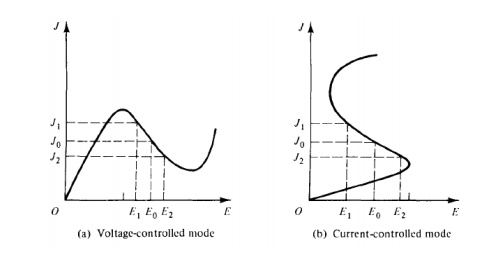Two-Valley Model Theory

According to the energy band theory of then-type GaAs, a high-mobility lower valley is separated by an energy of 0.36 eV from a low-mobility upper valleyWhen the applied electric field is lower than the electric field of the lower valley (£ < Ec), no electrons will transfer to the upper valley as show in Fig. 7-2-S(a).When the applied electric field is higher than that of the lower valley and lower than that of the upper valley (Ec < E < Eu), electrons will begin to transfer to the upper valley as shown in Fig. 7-2-S(b).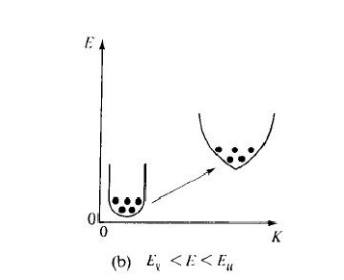And when the applied electric field is higher than that of the upper valley (Eu < E), all electrons will transfer to the upper valley as shown in Fig. 7-2-S(c).If electron densities in the lower and upper valleys are nc and nu , the conductivity of the n -type GaAs isWhen a sufficiently high field E is applied to the specimen, electrons are accelerated and their effective temperature rises above the lattice temperature. Furthermore, the lattice temperature also increases. Thus electron density n and mobility f-L are both functions of electric field E. Differentiation of Eq. (7-2-2) with respect toE yields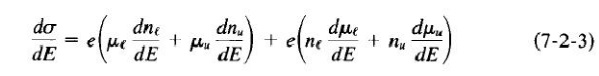If the total electron density is given by n = nt + nu and it is assumed that f.Le and /Lu are proportional to EP, where p is a constant, thenClearly, for negative resistance, the current density J must decrease with increasing field E or the ratio of dJ!dE must be negative. Such would be the case only if the right-hand term of Eq. (7-2-9) is less than zero. In other words, the condition for negative resistance is7. AVALANCE TRANSIT TIME DEVICES:

Avalanche transit-time diode oscillators rely on the effect of voltage breakdown across a reverse-biased p-n junction to produce a supply of holes and electrons. Ever since the development of modern semiconductor device theory scientists have speculated on whether it is possible to make a two-terminal negative-resistance device.

The tunnel diode was the first such device to be realized in practice. Its operation depends on the properties of a forward-biased p-njunction in which both the p and n regions are heavily doped. The other two devices are the transferred electron devices and the avalanche transit-time devices. In this chapter the latter type is discussed.

The transferred electron devices or the Gunn oscillators operate simply by the application of a de voltage to a bulk semiconductor. There are no p-n junctions in this device. Its frequency is a function of the load and of the natural frequency of the circuit. The avalanche diode oscillator uses carrier impact ionization and drift in the high-field region of a semiconductor junction to produce a negative resistance at microwave frequencies.

The device was originally proposed in a theoretical paper by Read in which he analyzed the negative-resistance properties of an idealized n+p- i-p+ diode. Two distinct modes of avalanche oscillator have been observed. One is the IMPATT mode, which stands for impact ionization avalanche transit-time operation. In this mode the typical dc-to-RF conversion efficiency is 5 to 10%, and frequencies are as high as 100 GHz with silicon diodes.

The other mode is the TRAPATT mode, which represents trapped plasma avalanche triggered transit operation. Its typical conversion efficiency is from 20 to 60%. Another type of active microwave device is the BARITT (barrier injected transit-time) diode . It has long drift regions similar to those of IMPATT diodes. The carriers traversing the drift regions of BARITT diodes, however, are generated by minority carrier injection from forward-biased junctions rather than being extracted from the plasma of an avalanche region. Several different structures have been operated as BARITT diodes, such as p-n-p, p-n-v-p, p-n-metal, and metal-nmetal. BARITT diodes have low noise figures of 15 dB, but their bandwidth is relatively narrow with low output power.

8. IMPATT AND TRAPATT DIODE:

Physical Structures

A theoretical Read diode made of ann+ -p-i-p+ or p+ -n-i-n+ structure has been analyzed. Its basic physical mechanism is the interaction of the impact ionization avalanche and the transit time of charge carriers. Hence the Read-type diodes are called IMPATT diodes. These diodes exhibit a differential negative resistance by two effects:

1)The impact ionization avalanche effect, which causes the carrier current lo(t) and the ac voltage to be out of phase by 90°

2) The transit-time effect, which further delays the external current l,(t) relative to the ac voltage by 90°

The first IMPATT operation as reported by Johnston et al.  in 1965, however, was obtained from a simple p-n junction. The first real Read-type IMPATT diode was reported by Lee et al. , as described previously.

From the small-signal theory developed by Gilden  it has been confirmed that a negative resistance of the IMPATT diode can be obtained from a junction diode with any doping profile.

Many IMPATT diodes consist of a high doping avalanching region followed by a drift region where the field is low enough that the carriers can traverse through it without avalanching.

The Read diode is the basic type in the IMPATT diode family. The others are the one-sided abrupt p-n junction, the linearly graded p-n junction (or double-drift region), and the p-i-n diode, all of which are shown in Fig. 8-2-1.

The principle of operation of these devices, however, is essentially similar to the mechanism described for the Read diode.

Negative Resistance

Small-signal analysis of a Read diode results in the following expression for the real part of the diode terminal impedance :The variation of the negative resistance with the transit angle when w > Wr is plotted in Fig. 8-2-2. The peak value of the negative resistance occurs near () = 7T. For transit angles larger than 7T and approaching 37T /2, the negative resistance of the diode decreases rapidly. For practical purposes, the Read-type IMPATT diodes work well only in a frequency range around the 7T transit angle. That is,Power Output and Efficiency

For a uniform avalanche, the maximum voltage that can be applied across the diode is given bywhere

Lis the depletion length

Em is the maximum electric field.

This maximum applied voltage is limited by the breakdown voltage. Furthermore, the maximum current that can be carried by the diode is also limited by the avalanche breakdown process, for the current in the space-charge region causes an increase in the electric field. The maximum current is given byIt is interesting to note that this equation is identical to Eq. (5-1-60) of the powerfrequency limitation for the microwave power transistor. The maximum power that can be given to the mobile carriers decreases as 1/ f. For silicon, this electronic limit is dominant at frequencies as high as 100 GHz. The efficiency of the IMPATT diodes is given by9. PARAMETRIC DEVICES:

Parametric Amplifiers

In a super heterodyne receiver a radio frequency signal may be mixed with a signal from the local oscillator in a nonlinear circuit (the mixer) to generate the sum and difference frequencies.

In a parametric amplifier the local oscillator is replaced by a pumping generator such as a reflex klystron and the nonlinear element by a time varying capacitor such as a varactor diode (or inductor) as shown in Fig.

In Fig. 8-5-2, the signal frequency !s and the pump frequency f, are mixed in the nonlinear capacitor C. Accordingly, a voltage of the fundamental frequencies !s andf, as well as the sum and the difference frequencies mfp ± nfs appears across C.

If a resistive load is connected across the terminals of the idler circuit, an output voltage can be generated across the load at the output frequency fa . The output circuit, which does not require external excitation, is called the idler circuit.

The output (or idler) frequency fa in the idler circuit is expressed as the sum and the difference frequencies of the signal frequency fsand the pump frequency fp,. That is,

where m and n are positive integers from zero to infinity.

If fo > fs , the device is called a parametric up-converter. Conversely, if fo < fs, the device is known as a parametric down-converter.Parametric up-converter.

A parametric up-converter has the following properties:

1)    The ouptut frequency is equal to the sum of the signal frequency and the pump frequency.

2)    There is no power flow in the parametric device at frequencies other than the

signal, pump, and output frequencies.

Power Gain.

When these two conditions are satisfied, the maximum power gain of a parametric up-converter  is expressed asMoreover, Rd is the series resistance of a p-n junction diode and yQ is the figure of merit for the nonlinear capacitor.Noise Figure.

One advantage of the parametric amplifier over the transistor amplifier is its low-noise figure because a pure reactance does not contribute thermal noise to the circuit. The noise figure F for a parametric up-converter  is given byBandwidth.

The bandwidth of a parametric up-converter is related to the gain-degradation factor of the merit figure and the ratio of the signal frequency to the output frequency. The bandwidth equation  is given byIf fol is = 10 and y = 0.2, the bandwidth (BW) is equal to 1.264.

Parametric down-converter.

The down-conversion gain (actually a loss) is given byNegative-resistance parametric amplifier.

If a significant portion of power flows only at the signal frequency Is , the pump frequency fp , and the idler frequency j; , a regenerative condition with the possibility of oscillation at both the signal frequency and the idler frequency will occur.

The idler frequency is  fi = fp - fs

When the mode operates below the oscillation threshold, the device behaves as a bilateral negative-resistance parametric amplifier.

Power Gain.

The output power is taken from the resistance R; at a frequency j; , and the conversion gain from Is to j;  is given byNoise Figure.

The optimum noise figure of a negative-resistance parametric amplifier  is expressed asThe optimum noise figure of a negative-resistance parametric amplifier  is expressed as

Bandwidth.

The maximum gain bandwidth of a negative-resistance parametric amplifier  is given byDegenerate parametric amplifier.

The degenerate parametric amplifier or oscillator is defined as a negative-resistance amplifier with the signal frequency equal to the idler frequency.

Power Gain and Bandwidth.

The power gain and bandwidth characteristics of a degenerate parametric amplifier are exactly the same as for the parametric up converter.Noise Figure.

The noise figures for a single-sideband and a double-sideband degenerate parametric amplifier  are given by, respectively,10. APPLICATION OF PARAMETRIC DEVICES

Applications

1.A positive input impedance

2.Unconditionally stable and unilateral

3.Power gain independent of changes in its source impedance

4.No circulator required

5.A typical bandwidth on the order of 5%

11. MICROWAVE MONOLITHIC INTEGRATED CIRCUITES

The metal-oxide-semiconductor field-effect transistor (MOSFET) is a four-terminal device. There are both n-channel and p-channel MOSFETs. The n-channel MOSFET consists of a slightly doped p -type semiconductor substrate into which two highly doped n + sections are diffused, as shown in Fig. 6-4-l.

These n + sections, which act as the source and the drain, are separated by about 0.5 f.Lm. A thin layer of insulating silicon dioxide (Si02) is grown over the surface of the structure. The metal contact on the insulator is called the gate. Similarly, the p -channel MOSFET is made of a slightly doped n -type semiconductor with two highly doped p +-type regions for the source and drain. The heavily doped polysilicon or a combination of silicide and polysilicon can also be used as the gate electrode. In practice, a MOSFET is commonly surrounded by a thick oxide to isolate it from the adjacent devices in a microwave integrated circuit. The basic device parameters of a MOSFET are as follows: L is the channel length, which is the distance between the two n+ -p junctions just beneath the insulator (say, 0.5 JLm), Z is the channel depth (say, 5JLm), dis the insulator thickness (say, 0.1 JLm), and r1 is the junction thickness of then+ section (say, 0.2 JLm).12. METERIALS AND FABRICATION TECHNIQUES

1. n-Channel Enhancement Mode (normally OFF). When the gate voltage is zero, the channel conductance is very low and it is not conducting. A positive voltage must be applied to the gate to form an n channel for conduction. The drain current is enhanced by the positive voltage. This type is called the enhancement-mode (normally OFF) n-channel MOSFET.

n-Channel Depletion Mode (normally ON). If an n channel exists at equilibrium (that is, at zero bias), a negative gate voltage must be applied to deplete the carriers in the channel. In effect, the channel conductance is reduced, and the device is turned OFF. This type is called the depletion-mode (normally ON) n-channel MOSFET.

-Channel Enhancement Mode (normally OFF). A negative voltage must be applied to the gate to induce a p channel for conduction. This type is called the enhancement-mode (normally OFF) p-channel MOSFET.

-Channel Depletion Mode (normally ON). A positive voltage must be applied to the gate to deplete the carriers in the channel for nonconduction. This type is called the depletion-mode (normally ON) p-channel MOSFET.Study Material, Lecturing Notes, Assignment, Reference, Wiki description explanation, brief detail
RF and Microwave Engineering : Microwave Semiconductor Devices : Microwave Semiconductor Devices |

Related Topics